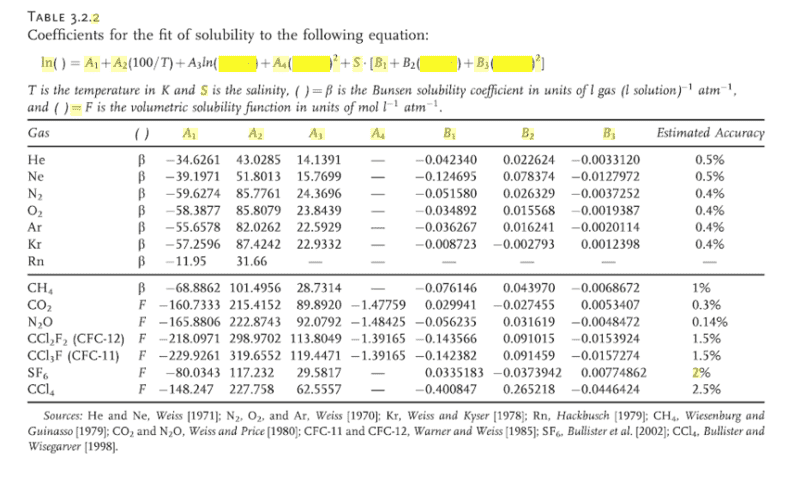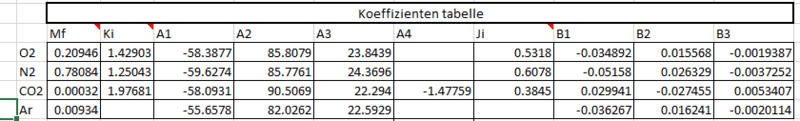# Gas Solubility in water - constants, and calculations

Solt
I can measure O2, Co2, TGP, and temperature, Want to calculate the rest gases, so N2, and Ar in my water.
There for i bulit up an axcel table. So i get to the point to calculate Bunsen contans on different Temperature.
I have this calculation:

ln(β)=exp(A1 + A2*(T/100) + A3*ln(T/100) + A4*(T/100) + S*(B1 + B2*(T/100) + B3*(T/100)^2

where β is in units of mL/L, and A# and B# are empirically derived least square fit constants, different for specifics gases.

Somehow, i wanted to find A1, A2, A3 and B1, B2, B3 constants for different Gases, but i dont finde.
I need for O2, CO2 , N2, Ar the constants.

Can somebody help where to finde, or how to get it ?

## Answers and Replies

Mentor
https://www.engineeringtoolbox.com/gases-solubility-water-d_1148.html

I think this will help. Be aware of STP (standard temperature and pressure) and the fact that Earth's atmosphere has different partial pressures for each atom/diatomic molecule. Which makes your job more difficult, IMO.

Note that temperature changes, higher for example, cause different results: gas A may have increased solubility at the higher temperature, gas B may have decreased solubility.

Last edited:
Homework Helper
There's a fat PDF here. Doesn't have all your coefficients but are you sure you need them all ? What's the application and what accuracy do you think you can achieve ?
Do you have access to aspen properties ?

Solt
Hi thanks for helping me!

So engineeringtoolbox.com i found before, but these are curves, and i cannot bild in a excel table. but Thanks Jim Mcnamara

Dear BvU

no im not so sure, but than how, i mean easily i can just measure:
O2 - Saturation %, or mg/L optica sensor
CO2 - Sensor, mg/L (i need it in saturation %)
TGP - Total gas Presseure in %

Some how i need to know N2 Saturation %. And i have a PSA, whats make the oxygenation in our Fischfarm.
What i know PSA makes 94% pure O2 (the rest 6% i dont know, but Throught our LHO (low head Oxigeniation ) system its get to our water.
If the N2 saturation goes over100, i have a problem, and if N2 saturation gets over 103% fish stop to eat, and die.
I need to be sure, thats never happened, so i need to measure whats going on.

So i thought, i know Temperature,
I know P(barometric)
i know salinity
And Saturation % of TGP, CO2, O2, lets calcculate the rest if its possible.
if you have easier solution i will be happy : )

Now i so far
I calculate for all the gases Busen Solubility coefficient, for temperauter, and salinityThan i can calculate partial pressure of gases
Except Argon i found Dissolved gas concentration constant
than i can calculate, partial gas pressure in gas form
what i can use for saturation (for all what i can measure, i can calculate
Than back from total gas pressure, and the partial gas pressure, i can calculate the rest gas pressure
and from that with temperature i can calculate saturatioon %, and also mg/L for N2

#### Attachments

Homework Helper
Can't follow you now. The table you post has all the gases you mention, so you can calculate all the saturation pressures. For non-equilibrium you will have to rely on the actual measurements. You could estimate the ones you can't measure by scaling down their saturation in proportion to that of a suitable measured gas.

Solt
you mean this?

%sat.N2 = [%sat.Total gas – (20,946*%sat.O2)/100]*100/79,054
Knowing that in Total gas you have: 20,946%O2 and 78,09%N2. The reste is composed of: Argon (0.93%) and CO2 (0.03%).

i have a feeling its not really works, while i have different gas componenets in PSA.

Solt
https://www.engineeringtoolbox.com/gases-solubility-water-d_1148.html

I think this will help. Be aware of STP (standard temperature and pressure) and the fact that Earth's atmosphere has different partial pressures for each atom/diatomic molecule. Which makes your job more difficult, IMO.

Note that temperature changes, higher for example, cause different results: gas A may have increased solubility at the higher temperature, gas B may have decreased solubility.

Yes i found these curves, but these curves i want to build in my calculator-thanks

Solt
So thanks for helping me, i show you what i mean
in the excel the first two tabel is important, the first is a calculator for the future
The second is the background

I made this, from M.B. Timmons /J.M.Ebeling Recirculating Aquaculture Book i found the calculations, and also the constants.Where
Mf -(some times he use Xi ) mole fraction
Ki - Ratio of the molacular weight to volume
mg/mL
A1
A2
A3
A4 (he dosent use at all)
Ji -Dissolved gas concetration constant in liquid
And B - for salinity he odesnt use and calcualte at all - thats what i want to build in

From that i can calculate the Busen coesfficient at different Temperature Tis in K, (so absolut)
β=exp(A1 + A2*(T/100) + A3*ln(T/100)

Pil=(Cmeas/β)*Ji
Where
Pil - Partial Pressure of gas in liquid
Cmeas - measured concentration level in mg/L

Knowing
Pbp - Barometric pressure
Pbp==10^(2.880814-(h/19748.2))
h - is above sea level
and
Pwv - water vapor pressure
Pwv=4.7603*(EXP(0.0645*T))
A0 = 4.7603, if Temperature used in °C

With thoose i can calculate
Pg - Partial Gas Pressure in gas form
Pg=Mf(Pbp-Pwv)

From that i can calculate from different gases all the saturation % what i measure in mg/L
Sat%=(Pil/Pg)*100

So frist step i have all my measurements what i need and i can calculate from measurements what i need to

Ptg sat% - Total gas Pressure - measured as saturation
Pil O2 - calculated from measured mg/L
Pil CO2 - calculated from measured mg/L
Pwv - Calculated from temperature
knowing that
Ptg=PilO2+PilN2+PilCO2+Pwv

Pil N2= Ptg-(PilO2+PilCO2+Pwv)
Pg N2 i can calculate
and
NO2 sat% =(PilN2/Pg N2)*100
so i have it.

My problem is there, Argon is missing from the calculation, ewhats also 0.93%, so would be nice to have
i dont finde A1, A2, A3 for Argon..or if i find, in the same table for known A1, A2, A3 is totally different (O2, CO2, N2) from Timmons given datas.

i want to bulid in the salinity, but somehow also totally different numbers and constants are given if i finde.

Thanks to take your time if you read it up to here.

#### Attachments

Solt
Can't follow you now. The table you post has all the gases you mention, so you can calculate all the saturation pressures. For non-equilibrium you will have to rely on the actual measurements. You could estimate the ones you can't measure by scaling down their saturation in proportion to that of a suitable measured gas.
Sorry, now i made the excel, and the calculations vlearer i hope
Down